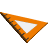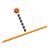You are here: Start » AVL.NET » Geometry 2D Distance Metrics

# Geometry 2D Distance Metrics

Icon Name DescriptionCircleToCircleDistance Measures the distance between two circles.PointSequenceDistances Measures the distances between consecutive points of a point sequence.PointToArcDistance Measures the distance between a point and an arc.PointToCircleDistance Measures the distance between a point and a circle.PointToLineDistance Measures the distance between a point and a line.PointToLineDistance_Oriented Measures the signed distance between a point and a line with direction.PointToPointDistance Measures the distance between two points.PointToSegmentDistance Measures the distance between a point and a segment.SegmentToSegmentDistance Measures the distance between two segments.